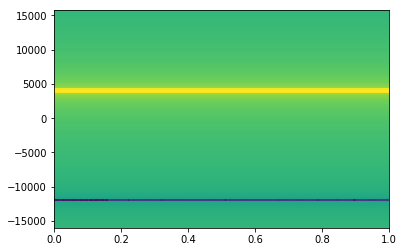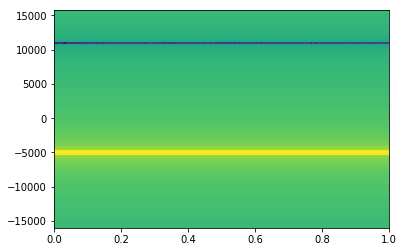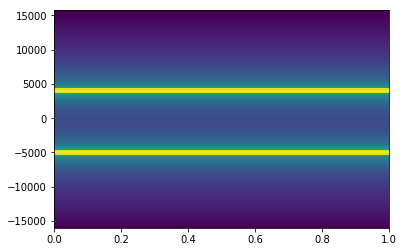In :
%matplotlib inline
import matplotlib
import matplotlib.pyplot as plt
import numpy as np
from scipy import signal
import IPython
import matplotlib as mpl
mpl.rcParams['figure.figsize'] = (14,8)

In :
SR=32000

def tone(freq, samples):
return np.exp(2j*np.pi*freq/SR*np.arange(samples))

In :
four_kay = tone(4000, SR)
_ = plt.specgram(four_kay, NFFT=128, noverlap=32, Fs=SR)In :
minus_five_kay = four_kay * tone(-9000, SR)
_ = plt.specgram(minus_five_kay, NFFT=128, noverlap=32, Fs=SR)In :
_ = plt.specgram(four_kay + minus_five_kay, NFFT=128, noverlap=32, Fs=SR)